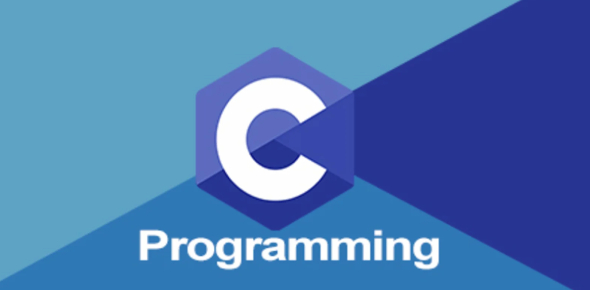# Quiz: C Programming MCQ Exam!

10 Questions | Attempts: 260
ShareSettingsAre you ready for this C Programming quiz? This quiz answers such questions as what the const feature can be applied to, the correct value to return to the operating system, what are not qualifiers in C, and the usual practice in declaring variables as volatile. Get ready to be tested on complex computer language. This quiz will test you about the C programming language. All the best.

• 1.
What are not qualifiers in C?
• A.

Static

• B.

Const

• C.

Volatile

• D.

None of the above

• 2.
Which of the following is not the usual practice in declaring variables as volatile?
• A.

Memory-mapped peripheral registers.

• B.

Global variables verified by an interrupt service routine.

• C.

Static variables.

• D.

• 3.
What is the output?     int main()     {        int i = 2*3 /4+4/ 4+3- 2+ 5 / 3;         printf("%d",i);         }
• A.

8

• B.

12

• C.

6

• D.

None of the above

• 4.
What is output? int  main() {           int x[ ] = { 1, 4, 3, 5, 1, 4 };        int *ptr, y;      ptr = x + 4;      y = ptr - x;        printf("%d",y);   }
• A.

3

• B.

5

• C.

4

• D.

1

• 5.
What is the output? int main() {    char *s="Abcat";    printf("%s",s+s-s);      }
• A.

BcatA

• B.

Cat

• C.

Bcat

• D.

Abca

• 6.
The “const” feature can be applied to?
• A.

An array argument

• B.

An identifier

• C.

An array

• D.

All of the above

• 7.
What is the correct value to return to the operating system upon the successful?
• A.

Null

• B.

1

• C.

-1

• D.

None of the above

• 8.
What is the output ?void f(char *);int main(int argc, char** argv){f("123");}void f(char a[]){if(a =='\0') return;f(a+1);f(a+1);printf("%c", a);}
• A.

321

• B.

333

• C.

332

• D.

321

• 9.
What is the output? int main(void)    {          static char food[] ="Yummy";       char *ptr;        ptr = food+strlen(food);        printf("%p",ptr);        return 0; }
• A.

Y

• B.

• C.

Unknown value

• D.

Run time error

• 10.
Int main(void){    int x = 2, y, z;    x *= 3 + 2;    x *= y = z = 4;    printf("%d \n", x);    x = y == z;    printf("%d \n", x);    return 0;}
• A.

40,1

• B.

40,40

• C.

40,4

• D.

None of the above

## Related TopicsBack to top
×

Wait!
Here's an interesting quiz for you.